# MathWorks Logo, Part Three, PDE Toolbox2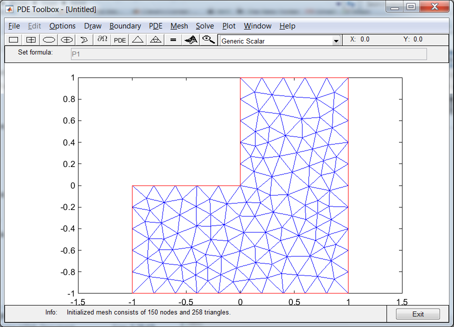The Partial Differential Equation Toolbox contains tools for the analysis of PDEs in two space dimensions and time. It is perhaps not surprising that one of the primary examples involves the L-shaped membrane.... read more >>

# MathWorks Logo, Part Two. Finite Differences2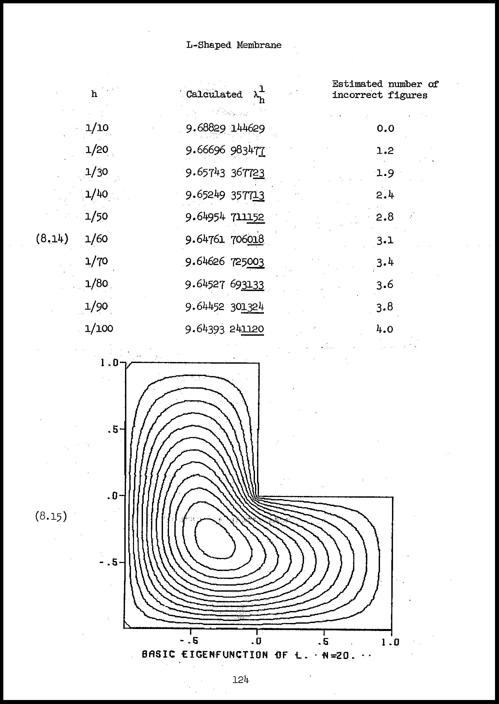After reviewing the state of affairs fifty years ago, I use classic finite difference methods, followed by extrapolation, to find the first eigenvalue of the region underlying the MathWorks logo.... read more >>

# MathWorks Logo, Part One. Why Is It L Shaped?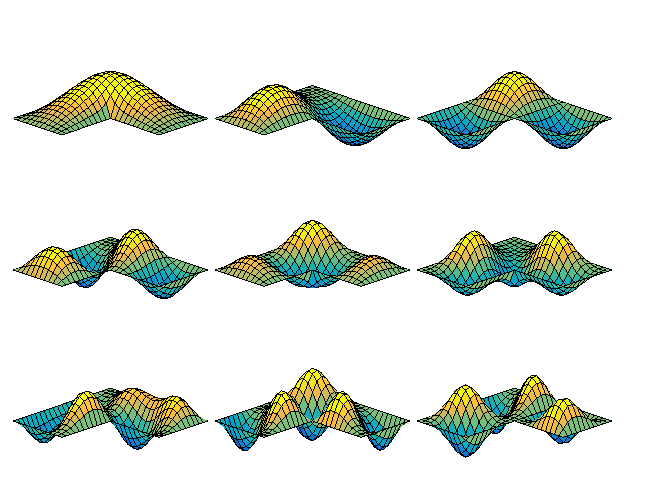MathWorks is the only company in the world whose logo satisfies a partial differential equation. Why is the region for this equation shaped like a capital letter L? ... read more >>

# Finite Fourier Transform Matrix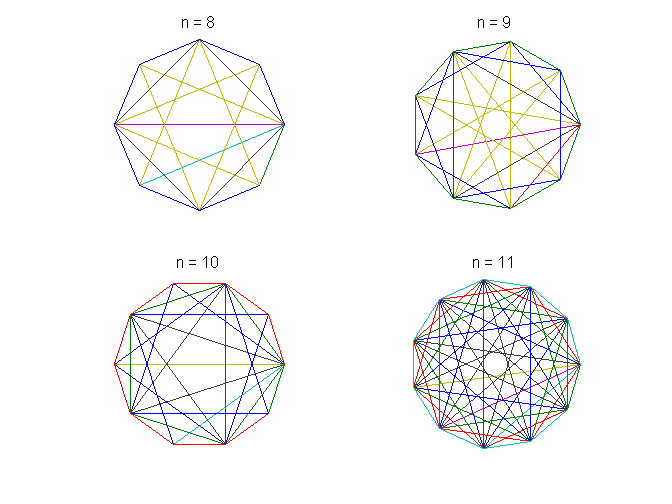This is the third in a series of posts on the finite Fourier transform. The Fourier matrix produces an interesting graphic and has a surprising eigenvalue distribution. ... read more >>

# Singular Value Analysis of Cryptograms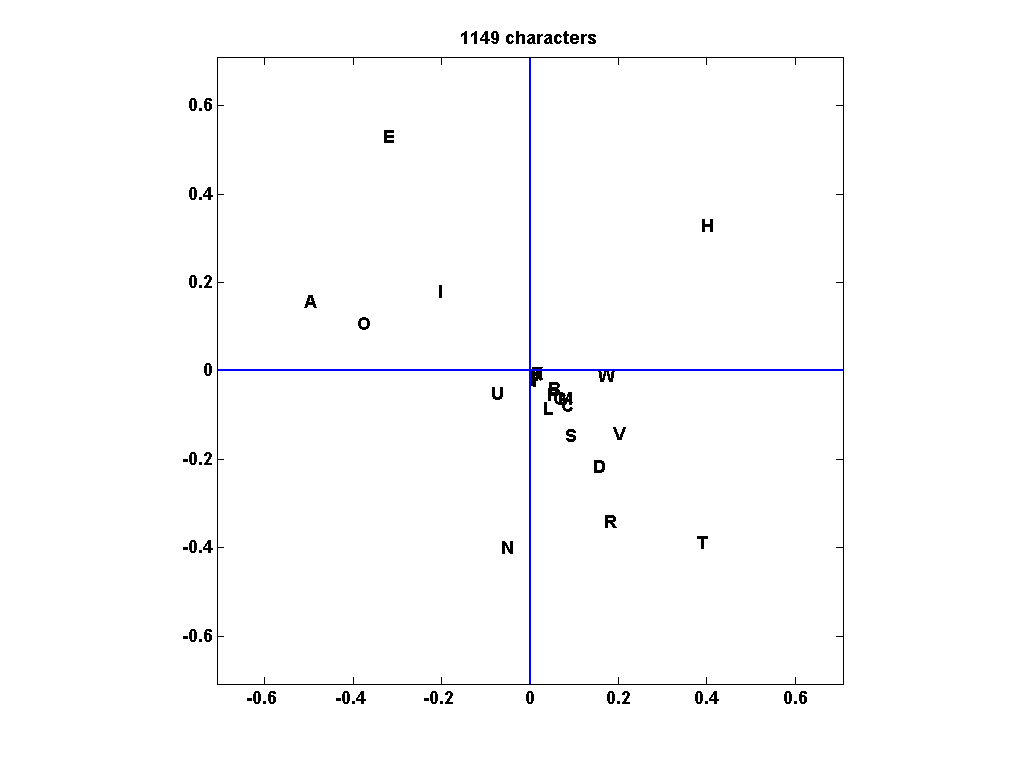The Singular Value Decomposition of the digram frequency matrix of a text coded with a simple substitution cypher can reveal information about the vowels and consonants in the text. ... read more >>

# Reverse Singular Value Decomposition2Employing a factorization based on the least significant singular values provides a matrix approximation with many surprisingly useful properties. This Reverse Singular Value Decomposition, RSVD, is also referred to as Subordinate Component Analysis, SCA, to distinguish it from Principal Component Analysis. ... read more >>

# Surprising SVD, Square Waves, and Pi2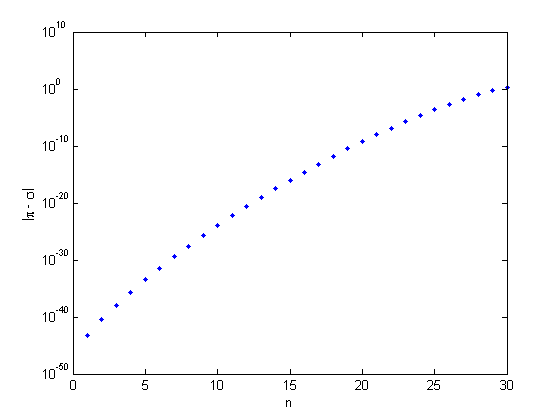I am surprised when many of the singular values of a nonsymmetric variant of the Hilbert matrix turn out to be nearly equal to $\pi$. The explanation involves the Fourier series for a square wave.... read more >>

# The Rosser Matrix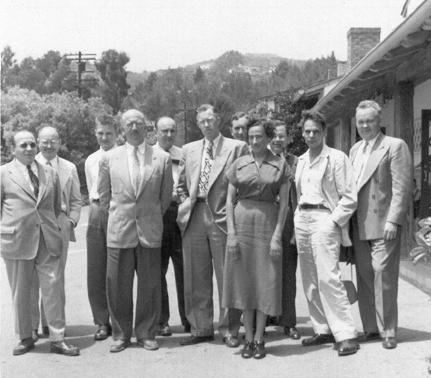The Rosser matrix is a classic matrix eigenvalue test problem.... read more >>

# Fiedler Companion Matrix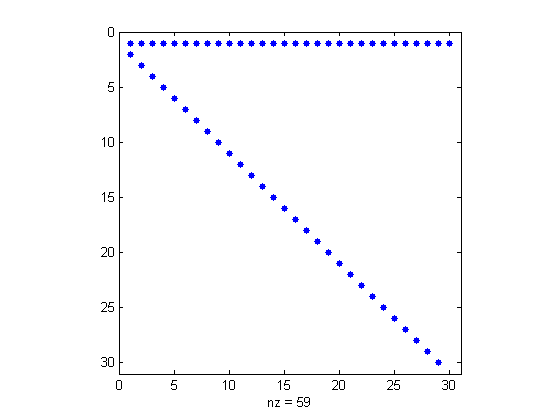The Fiedler companion matrix distributes the coefficients of a polynomial along the diagonals of an elegant pentadiagonal matrix whose eigenvalues are equal to the zeros of the polynomial.... read more >>

# eigshow, week 3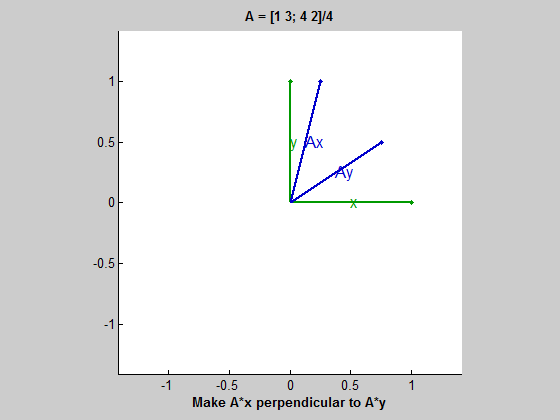An option in eigshow demonstrates SVD, the matrix singular value decomposition. The emphasis is on orthogonality.... read more >>